# 动手实现一个java中的散列表（HashTable）（文末福利）1 散列

2 散列函数

3 散列冲突

3.1 开放寻址法

3.2 链表法

4 手撕HashTable底层实现

5 *应用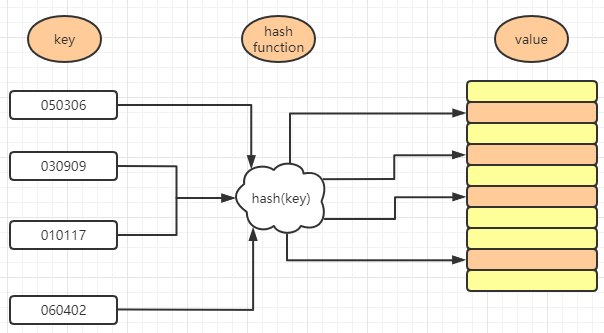``````int hash(String key) {
// 获取后两位字符
string lastTwoChars = key.substr(length-2, length);
// 将后两位字符转换为整数
int hashValue = Integer.parseInt(lastTwoChars);
return hashValue;
}``````

1. 散列函数计算得到的散列值是一个非负整数；
2. 如果 key1 = key2，那 hash(key1) == hash(key2)；
3. 如果 key1 ≠ key2，那 hash(key1) ≠ hash(key2)。

## 3.1 开放寻址法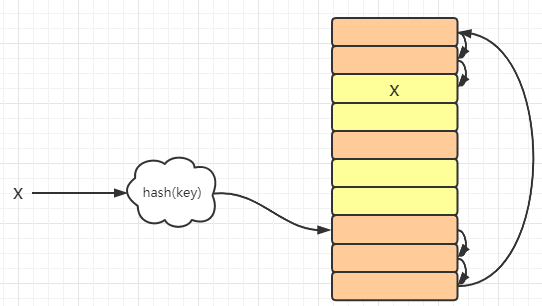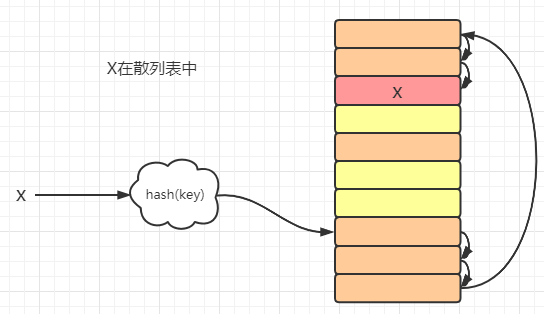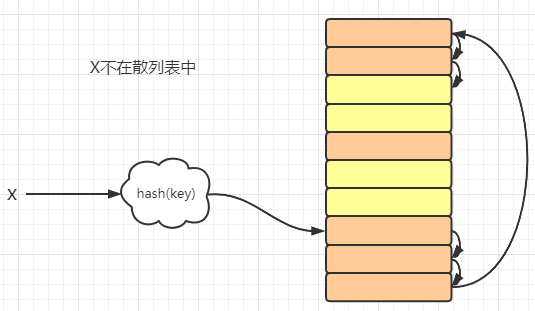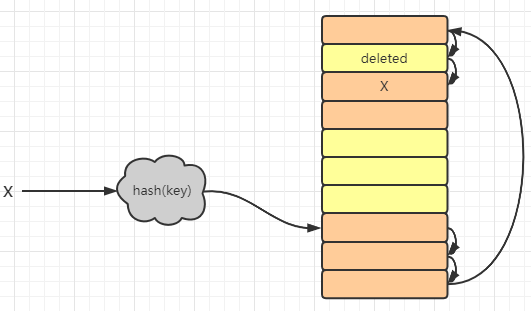## 3.2 链表法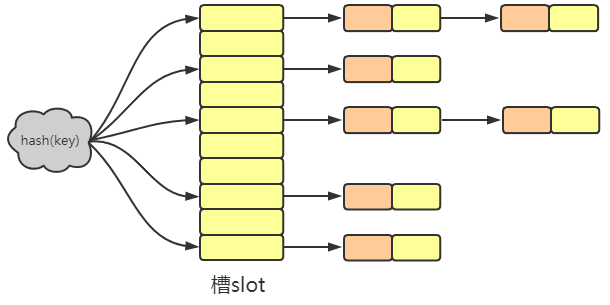``````public class HashTable<K, V> {

/**
*    容忍度上界
*/
private static final int upperTol = 10;

/**
*    容忍度下界
*/
private static final int lowerTol = 2;

/**
*    初始容量
*/
private static final int initCapacity = 7;

private TreeMap<K, V>[] hashtable;
// 长度（素数）
private int M;
// 已经存储了多少元素
private int size;

public HashTable(int M){
this.M = M;
size = 0;
hashtable = new TreeMap[M];
// 对每一个TreeMap进行初始化
for (int i = 0; i < M; i ++)
hashtable[i] = new TreeMap<>();
}

public HashTable(){
this(initCapacity);
}

}``````

``````private int hash(K key){
return key.hashCode() & 0x7fffffff % M;
}

public int getSize(){ return size; }``````

1. 根据key计算要插入的位置（对应一个TreeMap）
2. 如果TreeMap中已经存在这个key的话就进行value的更新
3. 如果TreeMap中不存在这个key的话则进行数据添加
4. 当元素过多时进行扩容操作
``````public void add(K key, V value){
// 计算要插入的位置
TreeMap<K, V> map = hashtable[hash(key)];
// key已存在
if (map.containsKey(key))
map.put(key, value);
else {
// key不存在
map.put(key, value);
size ++;

// 满足条件则扩容
if (size >= upperTol * M)
resize(2 * M);
}
}``````

1. 根据key计算要删除key对应的位置（对应一个TreeMap）
2. 判断是否包含这个key，存在的话则删除
3. 当元素过少时进行扩容操作
``````public V remove(K key){
// 计算要删除的位置
TreeMap<K, V> map = hashtable[hash(key)];
V ret = null;
// 存在则删除
if (map.containsKey(key)){
ret = map.remove(key);
size --;

// 一定情况下缩容
if (size < lowerTol * M && M / 2 >= initCapacity)
resize(M / 2);
}
return ret;
}``````

1. 根据key计算要进行修改的位置（对应一个TreeMap）
2. 存在该元素时则修改
``````public void set(K key, V value){
TreeMap<K, V> map = hashtable[hash(key)];
if (!map.containsKey(key))
throw new IllegalArgumentException(key + " doesn't exist!");
map.put(key, value);
}``````

查询操作：

``````public boolean contains(K key){
return hashtable[hash(key)].containsKey(key);
}

public V get(K key){
return hashtable[hash(key)].get(key);
}``````

``````private void resize(int newM){
TreeMap<K, V>[] newHashTable = new TreeMap[newM];
for (int i = 0; i < newM; i ++)
newHashTable[i] = new TreeMap<>();

int oldM = M;
// hash()函数需要新的M值
this.M = newM;
for (int i = 0; i < oldM; i ++){
TreeMap<K, V> map = hashtable[i];
for (K key: map.keySet())
newHashTable[hash(key)].put(key, map.get(key));
}

this.hashtable = newHashTable;
}

}``````

``````public static void main(String[] args) {
System.out.println("Pride and Prejudice");
ArrayList<String> words = new ArrayList<>();
// HashTable
startTime = System.nanoTime();
HashTable<String, Integer> ht = new HashTable<>(131071);
for (String word : words) {
if (ht.contains(word))
ht.set(word, ht.get(word) + 1);
else
}
for (String word: words)
ht.contains(word);
endTime = System.nanoTime();
time = (endTime - startTime)/1000000000.0;
System.out.println("HashTable: " + time);
}
}``````

执行用时：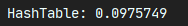HashMap的初始容量是16，并且容量都维持在n次方原因：性能考虑。只有2的n次幂的情况时最后一位二进制数才一定是1，这样能最大程度减少hash碰撞（hash值 & length-1）

HashMap对key的要求：不可变的，比如String被final修饰的。

HashMap存在线程安全问题，1.8之前使用头插法会产生环。我们可以使用ConcurrentHashMap来避免线程安全问题。

1.7之前：采用了分段锁机制来储存数据。在集合对象中储存一个segment数组，把集合的元素分为16个分段，每个分段上储存一个HashEntry数组及对应的链表，每个HashEntry数组就相当于一个HashMap。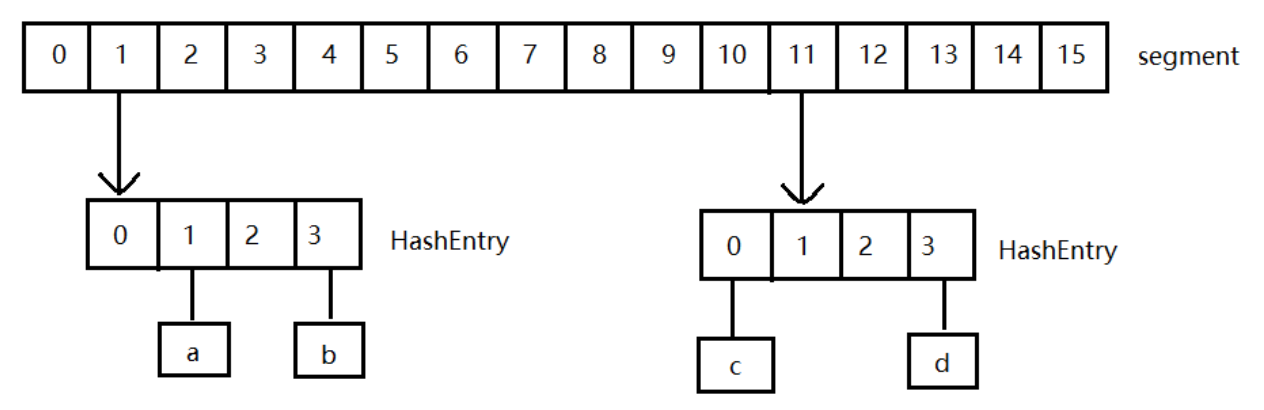1.8之后：利用CAS原理（比较交换原理）解决并发问题，其中有三个数：所存的值V，预期的值A，要变换成为的新值B。当A和V相同时，V会修改成B，否则不进行操作。这样就能较好的解决线程安全的问题。

-- 展开阅读全文 --

« 上一篇 07-24